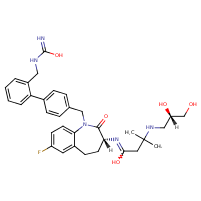Structure InformationCompound Identification

SMILES

CC(C)(CC(=O)N[C@@H]1CCC2=C(C=CC(F)=C2)N(CC2=CC=C(C=C2)C2=CC=CC=C2CNC(N)=O)C1=O)NC[C@H](O)CO

InChIKey

InChIKey=UBTRBYNKHSMPOD-XTEPFMGCSA-N

Formula

C33H40FN5O5

Mass

605.711